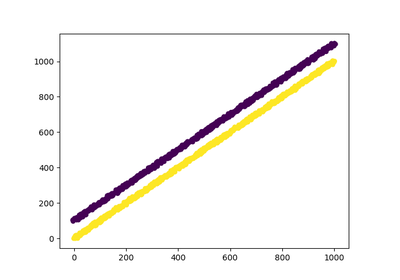# `sklearn.preprocessing`.FunctionTransformer¶

class `sklearn.preprocessing.``FunctionTransformer`(func=None, inverse_func=None, validate=True, accept_sparse=False, pass_y=’deprecated’, kw_args=None, inv_kw_args=None)[source]

Constructs a transformer from an arbitrary callable.

A FunctionTransformer forwards its X (and optionally y) arguments to a user-defined function or function object and returns the result of this function. This is useful for stateless transformations such as taking the log of frequencies, doing custom scaling, etc.

A FunctionTransformer will not do any checks on its function’s output.

Note: If a lambda is used as the function, then the resulting transformer will not be pickleable.

New in version 0.17.

Read more in the User Guide.

Parameters: func : callable, optional default=None The callable to use for the transformation. This will be passed the same arguments as transform, with args and kwargs forwarded. If func is None, then func will be the identity function. inverse_func : callable, optional default=None The callable to use for the inverse transformation. This will be passed the same arguments as inverse transform, with args and kwargs forwarded. If inverse_func is None, then inverse_func will be the identity function. validate : bool, optional default=True Indicate that the input X array should be checked before calling func. If validate is false, there will be no input validation. If it is true, then X will be converted to a 2-dimensional NumPy array or sparse matrix. If this conversion is not possible or X contains NaN or infinity, an exception is raised. accept_sparse : boolean, optional Indicate that func accepts a sparse matrix as input. If validate is False, this has no effect. Otherwise, if accept_sparse is false, sparse matrix inputs will cause an exception to be raised. pass_y : bool, optional default=False Indicate that transform should forward the y argument to the inner callable. kw_args : dict, optional Dictionary of additional keyword arguments to pass to func. inv_kw_args : dict, optional Dictionary of additional keyword arguments to pass to inverse_func.

Methods

 `fit`(X[, y]) Fit transformer by checking X. `fit_transform`(X[, y]) Fit to data, then transform it. `get_params`([deep]) Get parameters for this estimator. `inverse_transform`(X[, y]) Transform X using the inverse function. `set_params`(**params) Set the parameters of this estimator. `transform`(X[, y]) Transform X using the forward function.
`__init__`(func=None, inverse_func=None, validate=True, accept_sparse=False, pass_y=’deprecated’, kw_args=None, inv_kw_args=None)[source]
`fit`(X, y=None)[source]

Fit transformer by checking X.

If `validate` is `True`, `X` will be checked.

Parameters: X : array-like, shape (n_samples, n_features) Input array. self :
`fit_transform`(X, y=None, **fit_params)[source]

Fit to data, then transform it.

Fits transformer to X and y with optional parameters fit_params and returns a transformed version of X.

Parameters: X : numpy array of shape [n_samples, n_features] Training set. y : numpy array of shape [n_samples] Target values. X_new : numpy array of shape [n_samples, n_features_new] Transformed array.
`get_params`(deep=True)[source]

Get parameters for this estimator.

Parameters: deep : boolean, optional If True, will return the parameters for this estimator and contained subobjects that are estimators. params : mapping of string to any Parameter names mapped to their values.
`inverse_transform`(X, y=’deprecated’)[source]

Transform X using the inverse function.

Parameters: X : array-like, shape (n_samples, n_features) Input array. y : (ignored) X_out : array-like, shape (n_samples, n_features) Transformed input.
`set_params`(**params)[source]

Set the parameters of this estimator.

The method works on simple estimators as well as on nested objects (such as pipelines). The latter have parameters of the form `<component>__<parameter>` so that it’s possible to update each component of a nested object.

Returns: self :
`transform`(X, y=’deprecated’)[source]

Transform X using the forward function.

Parameters: X : array-like, shape (n_samples, n_features) Input array. y : (ignored) X_out : array-like, shape (n_samples, n_features) Transformed input.

## Examples using `sklearn.preprocessing.FunctionTransformer`¶Using FunctionTransformer to select columns